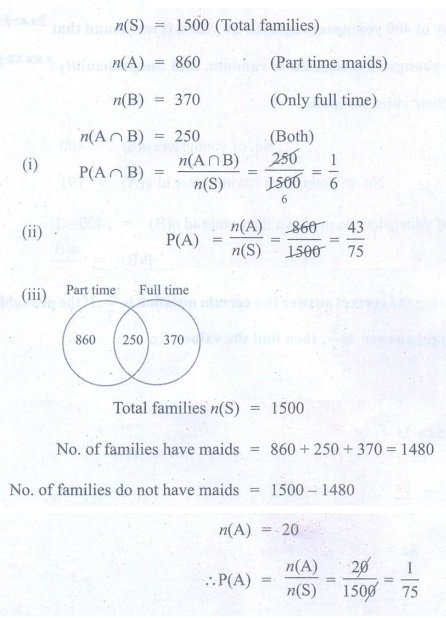Home | | Maths 9th std | Exercise 9.2: Types of Events

# Exercise 9.2: Types of Events

Maths : Probability: Book Back, Exercise, Example Numerical Question with Answers, Solution: Exercise 9.2: Types of Events - Probability

Probability: Types of Events

Exercise 9.2

1. A company manufactures 10000 Laptops in 6 months. Out of which 25 of them are found to be defective. When you choose one Laptop from the manufactured, what is the probability that selected Laptop is a good one.

Solution:2. In a survey of 400 youngsters aged 16-20 years, it was found that 191 have their voter ID card. If a youngster is selected at random, find the probability that the youngster does not have their voter ID card.

Solution:3. The probability of guessing the correct answer to a certain question is x/3. If the probability of not guessing the correct answer is x/5, then find the value of x .

Solution:4. If a probability of a player winning a particular tennis match is 0.72. What is the probability of the player loosing the match?

Solution:5. 1500 families were surveyed and following data was recorded about their maids at homesA family is selected at random. Find the probability that the family selected has

(i) Both types of maids (ii) Part time maids (iii)No maids

Solution:Exercise 9.2

1. 0.9975

2. 209/400

3. 15/8

4. 0.28

5.(i) 1/6 (ii) 43/75 (iii) 1/75

Tags : Numerical Problems with Answers, Solution | Probability | Maths , 9th Maths : UNIT 9 : Probability
Study Material, Lecturing Notes, Assignment, Reference, Wiki description explanation, brief detail
9th Maths : UNIT 9 : Probability : Exercise 9.2: Types of Events | Numerical Problems with Answers, Solution | Probability | Maths